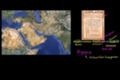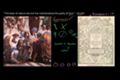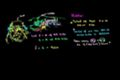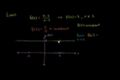Rachel

## Khan Academy on a Stick

### Math

•Arithmetic (261 videos)

So you're ready to have some arithmetic fun? You've come to the right spot! It's the first "official" math topic and chalked full of fun exercises and great videos which help you start your journey towards math mastery. We'll cover the big ones: addition, subtraction, multiplication, and division, of course. But we don't stop there, We'll get into negative numbers, absolute value, decimals, and fractions, too. Learning math should be fun, and we plan on having some with you. Ready to get started?

•Pre-algebra (383 videos)

No way, this isn't your run of the mill arithmetic. This is Pre-algebra. You're about to play with the professionals. Think of pre-algebra as a runway. You're the airplane and algebra is your sunny vacation destination. Without the runway you're not going anywhere. Seriously, the foundation for all higher mathematics is laid with many of the concepts that we will introduce to you here: negative numbers, absolute value, factors, multiples, decimals, and fractions to name a few. So buckle up and move your seat into the upright position. We're about to take off!

•Algebra basics (198 videos)

Algebra is a beautiful and important area of study with unlimited applications. One can spend a lifetime studying and exploring it (and some people do). If you're not one of them, and are looking to learn, review or practice the most core ideas in Algebra, you've found your home. This subject is ideal for anyone looking to prepare for a high school or college placement exam. It covers all of the foundational ideas in algebra and related topics in pre-algebra and geometry. If you're looking for more exhaustive coverage, then the Algebra I & II subjects may be better for you.

•Algebra I (589 videos)

Algebra is the language through which we describe patterns. Think of it as a shorthand, of sorts. As opposed to having to do something over and over again, algebra gives you a simple way to express that repetitive process. It's also seen as a "gatekeeper" subject. Once you achieve an understanding of algebra, the higher-level math subjects become accessible to you. Without it, it's impossible to move forward. It's used by people with lots of different jobs, like carpentry, engineering, and fashion design. In these tutorials, we'll cover a lot of ground. Some of the topics include linear equations, linear inequalities, linear functions, systems of equations, factoring expressions, quadratic expressions, exponents, functions, and ratios.

•Geometry (244 videos)

We are surrounded by space. And that space contains lots of things. And these things have shapes. In geometry we are concerned with the nature of these shapes, how we define them, and what they teach us about the world at large--from math to architecture to biology to astronomy (and everything in between). Learning geometry is about more than just taking your medicine ("It's good for you!"), it's at the core of everything that exists--including you. Having said all that, some of the specific topics we'll cover include angles, intersecting lines, right triangles, perimeter, area, volume, circles, triangles, quadrilaterals, analytic geometry, and geometric constructions. Wow. That's a lot. To summarize: it's difficult to imagine any area of math that is more widely used than geometry.

•Algebra II (308 videos)

Your studies in algebra 1 have built a solid foundation from which you can explore linear equations, inequalities, and functions. In algebra 2 we build upon that foundation and not only extend our knowledge of algebra 1, but slowly become capable of tackling the BIG questions of the universe. We'll again touch on systems of equations, inequalities, and functions...but we'll also address exponential and logarithmic functions, logarithms, imaginary and complex numbers, conic sections, and matrices. Don't let these big words intimidate you. We're on this journey with you!

•Trigonometry (89 videos)

Big, fancy word, right? Don't be fooled. Looking at the prefix, tri-, you could probably assume that trigonometry ("trig" as it's sometimes called) has something to do with triangles. You would be right! Trig is the study of the properties of triangles. Why is it important? It's used in measuring precise distances, particularly in industries like satellite systems and sciences like astronomy. It's not only space, however. Trig is present in architecture and music, too. Now you may wonder...how is knowing the measurement and properties of triangles relevant to music?? THAT is a great question. Maybe you'll learn the answer from us in these tutorials!

•Probability and statistics (172 videos)

We dare you to go through a day in which you never consider or use probability. Did you check the weather forecast? Busted! Did you decide to go through the drive through lane vs walk in? Busted again! We are constantly creating hypotheses, making predictions, testing, and analyzing. Our lives are full of probabilities! Statistics is related to probability because much of the data we use when determining probable outcomes comes from our understanding of statistics. In these tutorials, we will cover a range of topics, some which include: independent events, dependent probability, combinatorics, hypothesis testing, descriptive statistics, random variables, probability distributions, regression, and inferential statistics. So buckle up and hop on for a wild ride. We bet you're going to be challenged AND love it!

•Precalculus (165 videos)

You may think that precalculus is simply the course you take before calculus. You would be right, of course, but that definition doesn't mean anything unless you have some knowledge of what calculus is. Let's keep it simple, shall we? Calculus is a conceptual framework which provides systematic techniques for solving problems. These problems are appropriately applicable to analytic geometry and algebra. Therefore....precalculus gives you the background for the mathematical concepts, problems, issues and techniques that appear in calculus, including trigonometry, functions, complex numbers, vectors, matrices, and others. There you have it ladies and gentlemen....and introduction to precalculus!

•Differential calculus (155 videos)

How would you like to follow in the footsteps of Euclid and Archimedes? Would you like to be able to determine precisely how fast Usain Bolt is accelerating exactly 2 seconds after the starting gun? Differential calculus deals with the study of the rates at which quantities change. It is one of the two principle areas of calculus. The fathers of modern calculus, Isaac Newton and Gottfried Leibniz, independently formulated the fundamental theorem of calculus relating differentiation and integration. Differential calculus and integral calculus are connected by the fundamental theorem of calculus, which states that differentiation is the reverse process to integration.